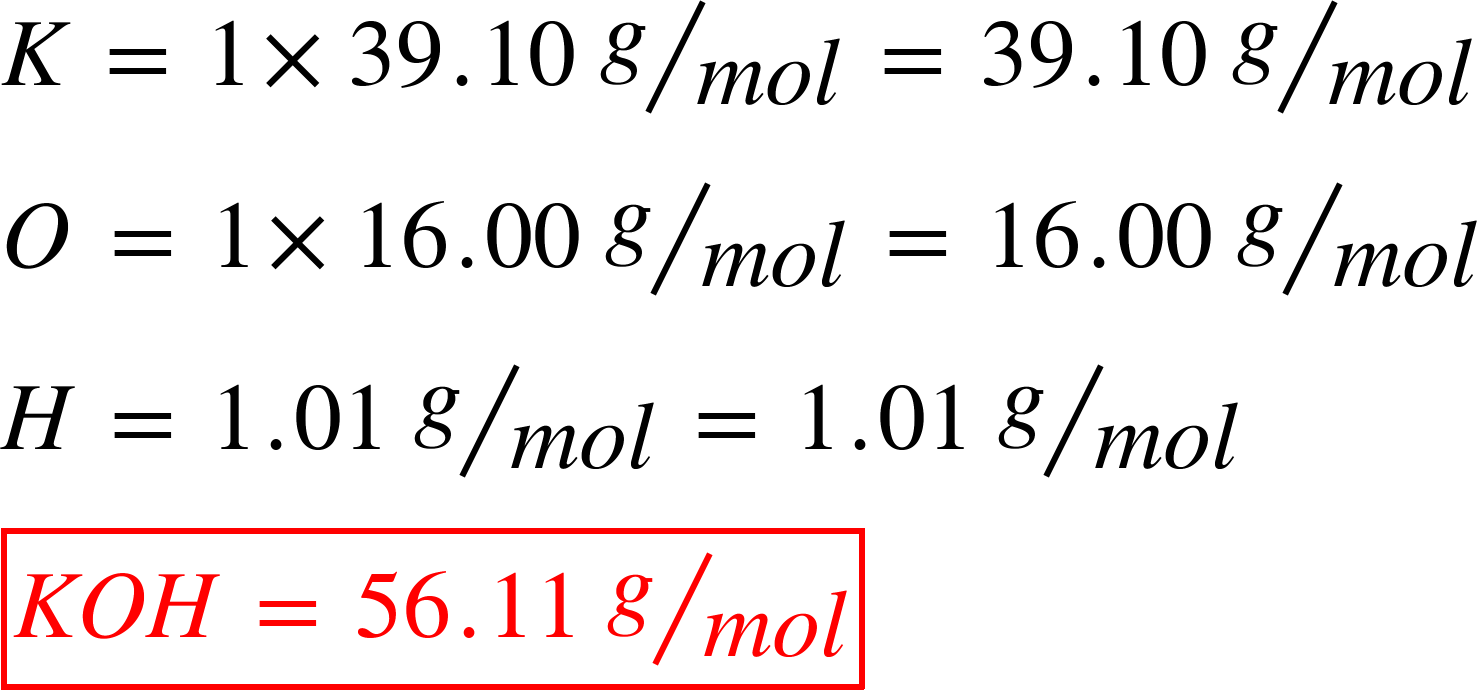# Problem: Determine the mass of each of the following:(a) 0.0146 mol KOH

###### FREE Expert Solution

A mole is a quantity of a substance that contains exactly 6.022x1023 atoms or molecules.

To convert moles to grams, we will be needing the molar mass of KOH.93% (428 ratings)###### Problem Details

Determine the mass of each of the following:

(a) 0.0146 mol KOH